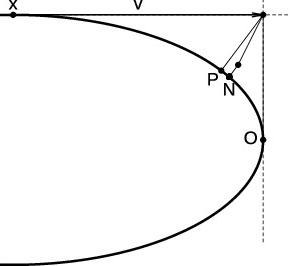# Newton Retraction as Approximate Geodesics on Submanifolds### Abstract

Efficient approximation of geodesics is crucial for practical algorithms on manifolds. Here we introduce a class of retractions on submanifolds, induced by a foliation of the ambient manifold. They match the projective retraction to the third order and thus match the exponential map to the second order. In particular, we show that Newton retraction (NR) is always stabler than the popular approach known as oblique projection or orthographic retraction: per Kantorovich-type convergence theorems, the superlinear convergence regions of NR include those of the latter. We also show that NR always has a lower computational cost. The preferable properties of NR are useful for optimization, sampling, and many other statistical problems on manifolds.

Type